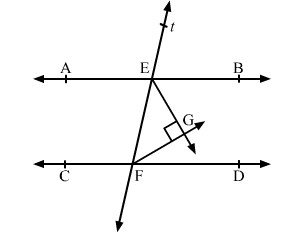# In the given figure, AB || CD and a transversal t cuts them at E and F respectively.

Question:

In the given figure, AB || CD and a transversal t cuts them at E and F respectively. If EG and FG are the bisectors of ∠BEF and ∠EFD respectively, prove that ∠EGF = 90°.Solution:

It is given that, AB || CD and is a transversal.

∠BEF + ∠EFD = 180°     .....(1)     (Sum of the interior angles on the same side of a transversal is supplementary)

EG is the bisector of ∠BEF.    (Given)

$\therefore \angle B E G=\angle G E F=\frac{1}{2} \angle B E F$

⇒ ∠BEF = 2∠GEF                .....(2)

Also, FG is the bisector of ∠EFD.    (Given)

$\therefore \angle \mathrm{EFG}=\angle \mathrm{GFD}=\frac{1}{2} \angle \mathrm{EFD}$

⇒ ∠EFD = 2∠EFG                .....(3)

From (1), (2) and (3), we have

2∠GEF + 2∠EFG = 180°

⇒ 2(
∠GEF + ∠EFG) = 180°

⇒
∠GEF + ∠EFG = 90°            .....(4)

In ∆EFG,

∠GEF + ∠EFG + ∠EGF = 180°          (Angle sum property)

⇒ 90° + ∠EGF = 180°                         [Using (4)]

⇒ ∠EGF = 180° − 90° = 90°